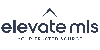Refinement

### 500 Results Found

Maximum number of listings showing. Try narrowing your search results.

#### Search Results

• Status Under contract - showing

#### 17160 E Goshawk Road, Colorado Springs, Colorado CO 80908-1628

Bedrooms

Total Baths

2,940 SqFt
5 Acresr46108956
39.079916,-104.634304Under Contract - Showing
• Status Active

#### 10350 Trail Creek Road, Divide, Colorado CO 80814

Total Baths

560 SqFt
45 Acresr46108956
39.031169,-105.257602Active
• Status Under contract - showing

#### 7 Mesa Lane, Colorado Springs, Colorado CO 80906-3122

Bedrooms

Total Baths

1,863 SqFt
0.320 Acresr46108956
38.796162,-104.850332Under Contract - Showing
• Status Under contract - showing

#### 1414 N Cascade Avenue, Colorado Springs, Colorado CO 80907-7405

Bedrooms

Total Baths

2,975 SqFt
0.262 Acresr46108956
38.854279,-104.824843Under Contract - Showing
• Status Active

#### 9127 Glitter Way, Colorado Springs, Colorado CO 80924-5375

Bedrooms

Total Baths

3,957 SqFt
0.234 Acresr46108956
38.965275,-104.709384Active
• Status Active

#### 5475 Castilian Villas Point, Colorado Springs, Colorado CO 80917-2612

Bedrooms

Total Baths

3,268 SqFt
0.159 Acres\$9K
r46108956
38.910875,-104.734275Active
• Status Active

#### 101 N Tejon Street330, Colorado Springs, Colorado CO 80903-1429

Bedrooms

Total Baths

1,547 SqFtr46108956
38.835548,-104.822784Active
• Status Active

#### 13366 Savannah Falls Court, Peyton, Colorado CO 80831

Bedrooms

Total Baths

2,916 SqFt
0.192 Acres\$5K
r46108956
38.984653,-104.578685Active
• Status Active

#### 152 Clarksley Road, Manitou Springs, Colorado CO 80829

Bedrooms

Total Baths

2,807 SqFt
0.218 Acresr46108956
38.852933,-104.893763Active
• Status Active

#### 2205 Vista Drive, Larkspur, Colorado CO 80118

Bedrooms

Total Baths

2,107 SqFt
5.070 Acresr46108956
39.205206,-104.928127Active
{"action":"https:\/\/search.coloradonewhomespecialists.com\/idx\/results\/listings","hiddenInputs":"<input name=\"start\" type=\"hidden\" value=\"1\" \/>\n<input name=\"hp\" type=\"hidden\" value=\"800000\" \/>\n<input name=\"lp\" type=\"hidden\" value=\"600000\" \/>\n<input name=\"pt\" type=\"hidden\" value=\"1\" \/>\n<input name=\"idxID\" type=\"hidden\" value=\"b035\" \/>\n<input name=\"city[]\" type=\"hidden\" value=\"2079\" \/>\n<input name=\"city[]\" type=\"hidden\" value=\"2305\" \/>\n<input name=\"city[]\" type=\"hidden\" value=\"4254\" \/>\n<input name=\"city[]\" type=\"hidden\" value=\"6758\" \/>\n<input name=\"city[]\" type=\"hidden\" value=\"7542\" \/>\n<input name=\"city[]\" type=\"hidden\" value=\"60485\" \/>\n<input name=\"city[]\" type=\"hidden\" value=\"7632\" \/>\n<input name=\"city[]\" type=\"hidden\" value=\"8660\" \/>\n<input name=\"city[]\" type=\"hidden\" value=\"9780\" \/>\n<input name=\"city[]\" type=\"hidden\" value=\"9783\" \/>\n<input name=\"city[]\" type=\"hidden\" value=\"9915\" \/>\n<input name=\"city[]\" type=\"hidden\" value=\"10870\" \/>\n<input name=\"city[]\" type=\"hidden\" value=\"12081\" \/>\n<input name=\"city[]\" type=\"hidden\" value=\"12374\" \/>\n<input name=\"city[]\" type=\"hidden\" value=\"14124\" \/>\n<input name=\"city[]\" type=\"hidden\" value=\"14215\" \/>\n<input name=\"city[]\" type=\"hidden\" value=\"14355\" \/>\n<input name=\"city[]\" type=\"hidden\" value=\"59462\" \/>\n<input name=\"city[]\" type=\"hidden\" value=\"16730\" \/>\n<input name=\"city[]\" type=\"hidden\" value=\"16928\" \/>\n<input name=\"city[]\" type=\"hidden\" value=\"19016\" \/>\n<input name=\"city[]\" type=\"hidden\" value=\"19510\" \/>\n<input name=\"city[]\" type=\"hidden\" value=\"21190\" \/>\n<input name=\"city[]\" type=\"hidden\" value=\"25618\" \/>\n<input name=\"city[]\" type=\"hidden\" value=\"28186\" \/>\n<input name=\"city[]\" type=\"hidden\" value=\"31038\" \/>\n<input name=\"city[]\" type=\"hidden\" value=\"7\" \/>\n<input name=\"city[]\" type=\"hidden\" value=\"35244\" \/>\n<input name=\"city[]\" type=\"hidden\" value=\"35525\" \/>\n<input name=\"city[]\" type=\"hidden\" value=\"36117\" \/>\n<input name=\"city[]\" type=\"hidden\" value=\"36348\" \/>\n<input name=\"city[]\" type=\"hidden\" value=\"42074\" \/>\n<input name=\"city[]\" type=\"hidden\" value=\"42076\" \/>\n<input name=\"city[]\" type=\"hidden\" value=\"46280\" \/>\n<input name=\"city[]\" type=\"hidden\" value=\"49035\" \/>\n<input name=\"city[]\" type=\"hidden\" value=\"52548\" \/>\n<input name=\"city[]\" type=\"hidden\" value=\"52928\" \/>\n<input name=\"srt\" type=\"hidden\" value=\"tba\" \/>\n","maxPerPage":"0","per":"10","currentPage":1,"lastPage":50,"path":"https:\/\/search.coloradonewhomespecialists.com\/idx\/results\/listings?start=1&hp=800000&lp=600000&per=10&pt=1&idxID=b035&city%5B%5D=2079&city%5B%5D=2305&city%5B%5D=4254&city%5B%5D=6758&city%5B%5D=7542&city%5B%5D=60485&city%5B%5D=7632&city%5B%5D=8660&city%5B%5D=9780&city%5B%5D=9783&city%5B%5D=9915&city%5B%5D=10870&city%5B%5D=12081&city%5B%5D=12374&city%5B%5D=14124&city%5B%5D=14215&city%5B%5D=14355&city%5B%5D=59462&city%5B%5D=16730&city%5B%5D=16928&city%5B%5D=19016&city%5B%5D=19510&city%5B%5D=21190&city%5B%5D=25618&city%5B%5D=28186&city%5B%5D=31038&city%5B%5D=7&city%5B%5D=35244&city%5B%5D=35525&city%5B%5D=36117&city%5B%5D=36348&city%5B%5D=42074&city%5B%5D=42076&city%5B%5D=46280&city%5B%5D=49035&city%5B%5D=52548&city%5B%5D=52928&srt=tba"}The real estate listing information and related content displayed on this site is provided exclusively for consumers' personal, non-commercial use and may not be used for any purpose other than to identify prospective properties consumers may be interested in purchasing. Any offer of compensation is made only to Participants of the PPMLS. This information and related content is deemed reliable but is not guaranteed accurate by the Pikes Peak REALTOR® Services Corp.

Information Last Updated: Monday, October 3rd, 2022
Data services provided by IDX Broker